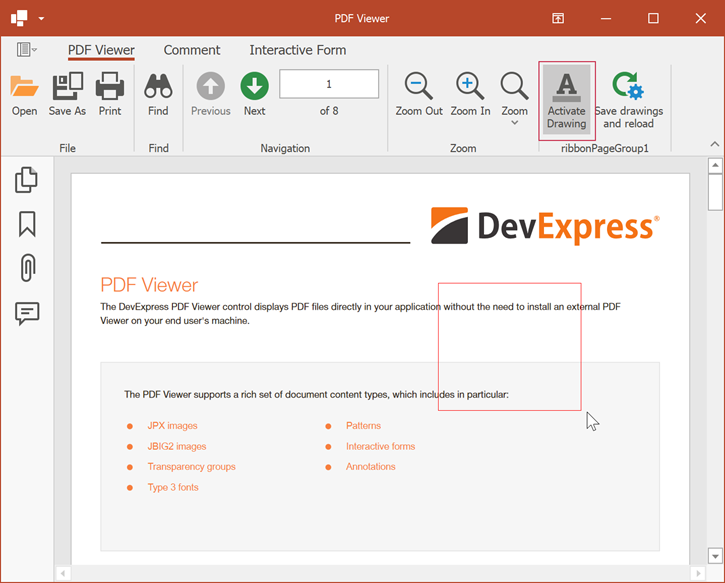# How to: Use Coordinates Obtained from a PDF Viewer to Draw Graphics

The following example demonstrates how to use coordinates obtained from a PDF Viewer to draw graphics content. Use the System.Drawing.Graphics class to draw graphics in the `Paint` event handler. The PDF Graphics API allows you to save graphics in a document.

Important

The Universal Subscription or an Office File API Subscription is required to use the PDF Document API in production code. Refer to the DevExpress Subscription page for pricing information.

This example does the following:

• Allows a user to select a rectangular area with the mouse.
• When the user releases the mouse button, it draws a red rectangle over this selection.
• Saves the created graphics to the PDF file.

The PDF Viewer has a custom Activate Drawing button that enables drawing mode.## Obtain Coordinates of the Selected Area

Handle the `MouseUp`, `MouseMove`, and `MouseDown` events to obtain the selected area coordinates. The PdfViewer.GetDocumentPosition method allows you to convert the retrieved coordinates to page coordinates. The `GraphicsCoordinates` class is used to save and restore these coordinates.

``````// This class is used to save
// and restore the selection area coordinates
class GraphicsCoordinates
{
public GraphicsCoordinates(int pageIndex, PdfPoint point1, PdfPoint point2) {
PageIndex = pageIndex;
Point1 = point1;
Point2 = point2;
}

public int PageIndex { get; }
public PdfPoint Point1 { get; }
public PdfPoint Point2 { get; }
public bool IsEmpty => Point1 == Point2;
}

List<GraphicsCoordinates> rectangleCoordinateList = new List<GraphicsCoordinates>();
GraphicsCoordinates currentCoordinates;

// This variable indicates whether the Drawing button
// is activated
bool ActivateDrawing = false;
// ...
void PdfViewer_MouseMove(object sender, MouseEventArgs e) {
if (currentCoordinates != null) {
UpdateCurrentRect(e.Location);
pdfViewer.Invalidate();
}
}
void pdfViewer1_MouseUp(object sender, MouseEventArgs e) {

// Convert the retrieved coordinates
// to the page coordinates
UpdateCurrentRect(e.Location);
if (currentCoordinates != null) {
if (!currentCoordinates.IsEmpty && ActivateDrawing)
// Add coordinates to the list
currentCoordinates = null;
}
}
void pdfViewer1_MouseDown(object sender, MouseEventArgs e) {
var position = pdfViewer.GetDocumentPosition(e.Location, true);
currentCoordinates = new GraphicsCoordinates(position.PageNumber - 1, position.Point, position.Point);
}

void UpdateCurrentRect(Point location)
{
if (rectangleCoordinateList != null)
{
var documentPosition = pdfViewer.GetDocumentPosition(location, true);
if (currentCoordinates.PageIndex == documentPosition.PageNumber - 1)

currentCoordinates = new GraphicsCoordinates(currentCoordinates.PageIndex, currentCoordinates.Point1, documentPosition.Point);
}
}
``````

## Handle the Paint Event to Draw Graphics

In the PdfViewerControl.Paint event handler, check if the Drawing button is activated and use the obtained coordinates to draw a rectangle. The PdfViewer.GetClientPoint(PdfDocumentPosition) method allows you to convert the `PdfDocumentPosition` instance to the PointF object.

The code below paints graphics in the UI control, separate from the document content. Any painted graphics does not persist within the document.

``````private void activateDrawingButton_ItemClick(object sender, DevExpress.XtraBars.ItemClickEventArgs e)
{
// Change the activation indicator
ActivateDrawing = !ActivateDrawing;
pdfViewer.Invalidate();
}

void PdfViewer_Paint(object sender, PaintEventArgs e) {
if (ActivateDrawing)
{
foreach (var r in rectangleCoordinateList)
DrawImageRectangle(e.Graphics, r);
if (currentCoordinates != null)
DrawImageRectangle(e.Graphics, currentCoordinates);
}
}

void DrawImageRectangle(Graphics graphics, GraphicsCoordinates rect)
{
PointF start = pdfViewer.GetClientPoint(new PdfDocumentPosition(rect.PageIndex + 1, rect.Point1));
PointF end = pdfViewer.GetClientPoint(new PdfDocumentPosition(rect.PageIndex + 1, rect.Point2));
// Create a rectangle where graphics should be drawn
var r = Rectangle.FromLTRB((int)Math.Min(start.X, end.X), (int)Math.Min(start.Y, end.Y), (int)Math.Max(start.X, end.X), (int)Math.Max(start.Y, end.Y));

// Draw a rectangle in the created area
graphics.DrawRectangle(new Pen(Color.Red), r);
}
``````

## Draw Graphics into a Document and Save the Result

The graphics content created by the System.Drawing.Graphics class is not retained after the document is saved. Use the PdfDocumentProcessor and PdfGraphics instances to draw graphics content in the document and save the result.

The code sample below shows the `SaveDrawingAndReload` method that draws graphics content, saves the result, and reopens the PDF file. The method is executed on a button click.

``````private void saveGraphicsButton_ItemClick(object sender, DevExpress.XtraBars.ItemClickEventArgs e)
{
}

{
string fileName = pdfViewer.DocumentFilePath;
pdfViewer.CloseDocument();
using (PdfDocumentProcessor processor = new PdfDocumentProcessor())
{
// Load a document to the PdfDocumentProcessor instance
foreach (var rect in rectangleCoordinateList)
{
// Create a PdfGraphics object
using (PdfGraphics graph = processor.CreateGraphics())
{
PdfPage page = processor.Document.Pages[rect.PageIndex];
PdfRectangle pageCropBox = page.CropBox;
PdfPoint p1 = new PdfPoint(rect.Point1.X, pageCropBox.Height - rect.Point1.Y);
PdfPoint p2 = new PdfPoint(rect.Point2.X, pageCropBox.Height - rect.Point2.Y);

// Create a rectangle where graphics should be drawn
RectangleF bounds = RectangleF.FromLTRB(
(float)Math.Min(p1.X, p2.X), (float)Math.Min(p1.Y, p2.Y),
(float)Math.Max(p1.X, p2.X), (float)Math.Max(p1.Y, p2.Y));
// Draw a rectangle in the created area
graph.DrawRectangle(new Pen(Color.Red), bounds);

// Draw graphics content into a file
}
}
// Save the document
processor.SaveDocument(fileName);
}
rectangleCoordinateList.Clear();

// Open the document in the PDF Viewer